+
Common Core: S.ID.6c

# Linear Equation Algebra Review

##### Rating:
(9)
• (3)
• (0)
• (1)
• (3)
• (2)
Author: Sophia Tutorial
##### Description:

Calculate the slope and y-intercept of a given situation.

(more)
Tutorial
what's covered
This tutorial will review the basic algebra of linear equations. Our discussion breaks down as follows:

1. Linear Equations
1. Slope and Intercept In Practice
2. Slope and Intercept in Statistics
2. Using a Line to Obtain Points
3. Using Two Points to Obtain a Line
4. Establishing Variables

## 1. Linear Equations

As lines fit to data, figuring out the equations of those lines is the focus of this tutorial. You may recall the following linear equation:

formula
Slope-Intercept Form of a Linear Equation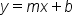In the above equation, y and x are variables. Now, x is recognized as the explanatory variable and y as the response variable. The other two values are numbers, and they represent something.

The value of m is called the slope. The slope is a rate of change. You may have heard several terms of rates of change, like miles per hour or meters per second or miles per gallon in a car. In general, it's an increase of 1 in x corresponds to an increase or decrease of m in y.

EXAMPLE

If the rate of change was 30 miles per gallon, that means that an increase of one gallon would correspond to an increase of 30 miles that you could travel.

The slope is calculated by taking the difference in y values divided by the difference in x values.

formula
Slope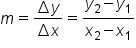The other value, b, in the equation is called the y-intercept. It's the value of y when x is 0. So the line will pass through the point (0, b) on the y-axis.

terms to know

Slope
The rate of change relating the increase or decrease in y to an increase of 1 in x.
y-intercept
The value of y when x = 0.

1a. Slope and Intercept in Practice

Let's show these terms in practice, in the graph below.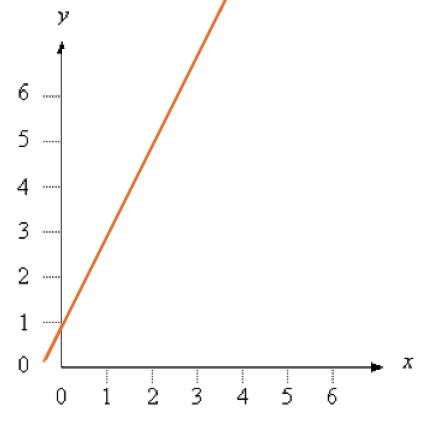To find the slope, what we have to do is find out the points that are on the line, and figure out by how much vertically this went up with an increase of 1 in x. Let consider how much it went up between 1 and 2 on the x-axis.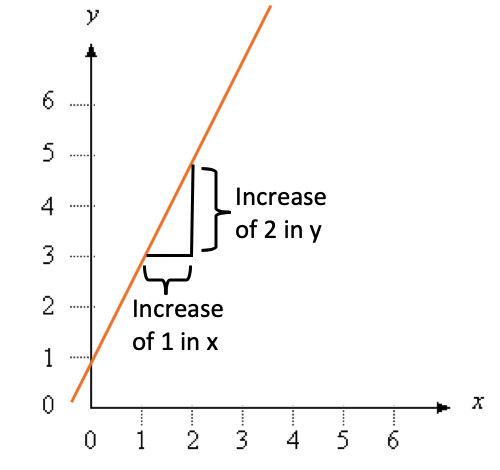As the graph shows an increase of 1 in x, there was an increase of 2 in y. So the slope, which explains the rate of change that relates the increase in y to an increase of 1 in x, would be 2.

Also, the y-intercept is the point where the line passes through the y-axis, or at (0,1). So the value of y when x is 0 is 1, which would be b in the linear equation.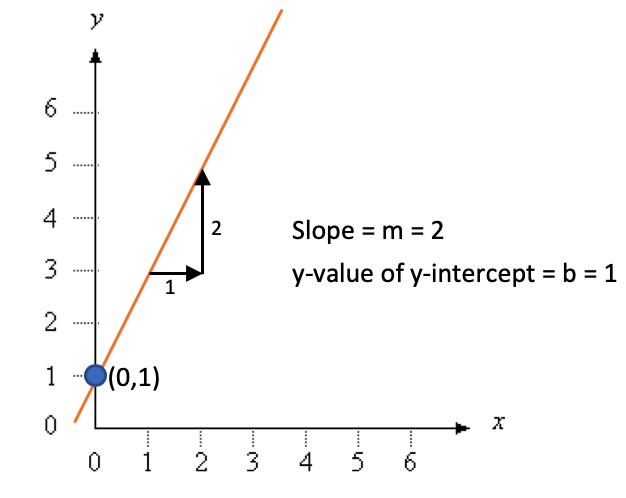1b. Slope and Intercept in Statistics

Below is the general linear equation compared to the formula used in statistics.

formula
Slope-Intercept Form of a Linear Equation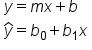You may notice that the order is flipped, but it is still telling us the same information and can be used to find the best-fit line.

• Instead of m, the slope becomes b1
• Instead of b, the y-intercept becomes b0
• Instead of y, we now have what is called y hat.

The variable y-hat is the notation for the prediction. There are values of y that are not predictions; they're actual data points. But because we're doing a best fit, this is our best guess as the value of y--it's a prediction. Anything with a hat is called a prediction.

## 2. Using a Line to Obtain Points

Suppose that you have a trend line and the equation is: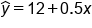We can use this equation to find the predicted y-coordinate, y-hat, when x equals 20. To solve this algebraically, all you have to do is substitute 20 in for x in the given equation:## 3. Using Two Points to Obtain a Line

Suppose we don't know the equation of a trend line, but we know that it passes through (4, 500) and (12, 900).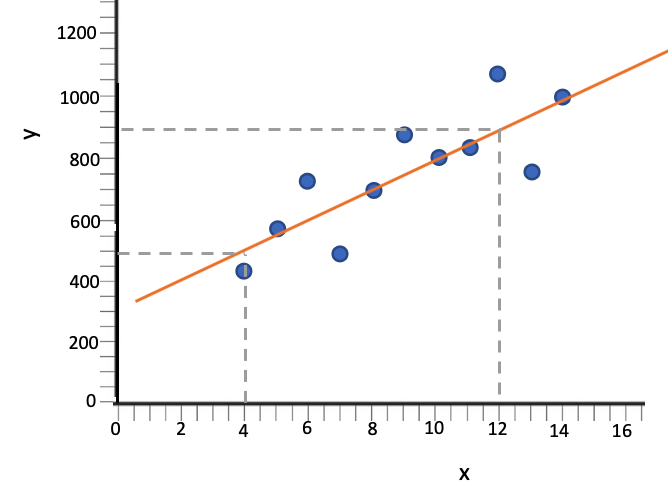To find the equation of that line, two pieces of information are needed: the slope and the y-intercept.

First, find the slope. You can see visually that from (4, 500) to (12, 900), it went up 8 in the x-direction. But it also actually went up 400 in the y-direction.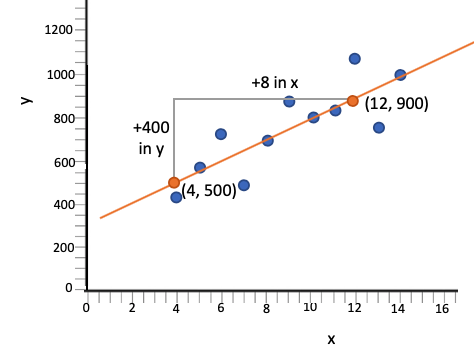Recall that slope is the difference in y values divided by the difference in x values. So a change of 400 in the y-direction divided by a change of 8 in the x-direction means that for every 1 increase in the x-direction, it actually went up 400 divided by 8, or 50 in the y. Therefore, the slope, b1, is 50.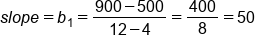To figure out the y-intercept, plug any (x, y) pair that is on the line into the equation, for this example, (12, 900). Put 12 temporarily in for x and 900 temporarily in for y-hat. We also know the slope, 50, so we can plug this value in for b1.This tells us that the y-intercept, b0 is 300. Plug in this value and the slope into the linear equation formula to get: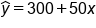## 4. Establishing Variables

One thing that's important to note is that the best-fit line will change if you switch the explanatory and response variables. That's why it's important to choose at the beginning which one is the explanatory verses which one is the response variable.

EXAMPLE

Slope is a rate of change. So if you take a look below, miles per gallon would be the rate of change in the example on the right. If you switched to put gallons on the y-axis and miles on the x-axis, the rate of change here would actually be measuring gallons per mile, which is a different number.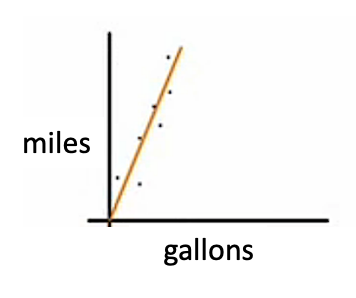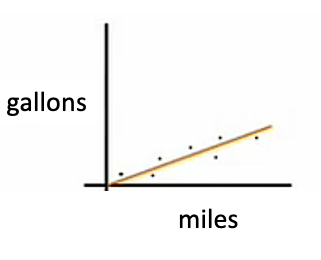If a car is getting an average of 20 miles per gallon that will actually only be one-twentieth gallons per mile. It's a different line.

One thing that's important to note, though, is that the value of the correlation coefficient is going to be the same for each of these two graphs, but the line itself is different, and that's why we need to choose which variable is the explanatory versus which one is the response.

summary
Fitting a line to data points on the scatter plot requires a bit of algebraic savvy. There are two parts to the equation of a line: the slope and the y-intercept. Linear equations involve figuring out the equations of those lines. The slope is a rate of change. It's how quickly the response variable y changes when the explanatory variable x increases by 1. The y-intercept is the value of y when x equals 0. In actual practice, you will want to put variable names and units attached to these, not just x's and y's.

Good luck!

Source: Adapted from Sophia tutorial by Jonathan Osters.

Terms to Know
Slope

The rate of change relating the increase or decrease in y to an increase of 1 in x.

y-intercept

The value of y when x = 0.

Formulas to Know
SlopeSlope-Intercept Form of a Linear EquationRating Header